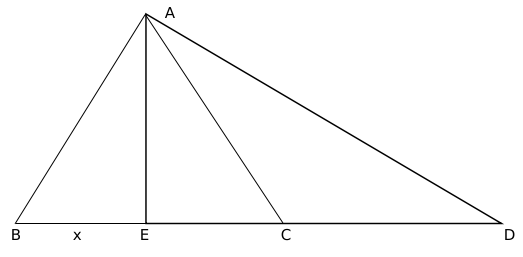Question 15

# $$ABC$$ is a triangle, $$\angle B=60^\circ, \angle C=45^\circ$$, BC is produced to / extended till D so that $$\angle ADB=30^\circ$$, then given $$\sqrt{3}=\frac{19}{11}$$ and $$BC \times CD=3^{4}\times 2^{3}\times\frac{11}{19}$$, what will be the square of the altitude from A to BC?

SolutionAE is altitude from A on BC. $$\angle\ AEC\ =\ 90^{\circ\ }$$

It is given,

$$\angle\ ABC\ =\ 60^{\circ\ },\ \angle\ ACB\ =\ 45^{\circ\ }$$

In triangle ABE,

AE = $$\sqrt{\ 3}x$$

As $$\angle\ EAC\ =\angle\ ECA$$, EC = $$\sqrt{\ 3}x$$

In triangle AED, $$\angle\ EAD\ =\ 60^{\circ\ }$$

Therefore, CD = $$3x-\sqrt{\ 3}x$$

It is given,

$$BC\ \cdot\ CD\ =\ \frac{3^4.2^3.11}{19}$$

$$x\left(\sqrt{\ 3}+1\right)\sqrt{\ 3}\left(\sqrt{\ 3}-1\right)x\ =\ \frac{3^4.2^3.11}{19}$$

$$x\ =\ \frac{3^2.2.11}{19}$$

AE = 9*2 = 18

$$AE^2$$ = 324